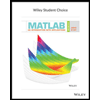# A sample of midterm grades for five students showed the following results: 72, 65, 82,90, 76. Which of the following statements are correct, and which should be challenged asbeing too generalized?a. The average midterm grade for the sample of five students is 77.b. The average midterm grade for all students who took the exam is 77.c. An estimate of the average midterm grade for all students who took the exam is 77.d. More than half of the students who take this exam will score between 70 and 85

Question

A sample of midterm grades for five students showed the following results: 72, 65, 82,
90, 76. Which of the following statements are correct, and which should be challenged as
being too generalized?
a. The average midterm grade for the sample of five students is 77.
b. The average midterm grade for all students who took the exam is 77.
c. An estimate of the average midterm grade for all students who took the exam is 77.
d. More than half of the students who take this exam will score between 70 and 85

Expert Solution

### Want to see the full answer?

Check out a sample Q&A hereStudents who’ve seen this question also like:MATLAB: An Introduction with Applications
6th Edition
ISBN: 9781119256830
Author: Amos Gilat
Publisher: John Wiley & Sons Inc